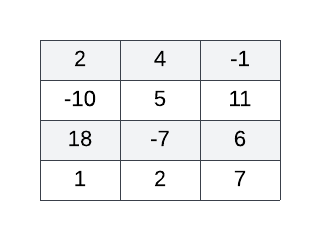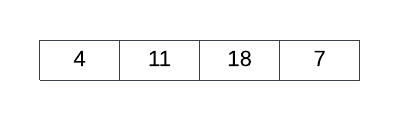Login or Create an Account to view question mark schemes, comment, and download a PDF of this test
Question 1[11 Marks]
An anagram is a word or phrase formed by rearranging the letters of another. For example, “listen” and “silent” are anagrams of one another.
Consider an array called ALPHA of size 26 initialised to contain only zeros. Also consider a function LTR2NUM() which accepts a single character and returns it's position in the English alphabet. For example, LTR2NUM('a') and LTR2NUM('z') return 1 and 26 respectively.
(a).
Given a Collection of letters called LETTERS, construct an algorithm to accumulate the total number of each type of letter in corresponding ALPHA index locations. For example, if the letter 'b' occurs 7 times in LETTERS then, once the algorithm has completed, ALPHA should equal 7.

(b).
Construct an algorithm that outputs True if every value in ALPHA is equal to zero

Assume now that the two algorithms from a) and b) were augmented to form functions called createAlpha() and isZero() respectively.
The function createAlpha() accepts as input a Collection of letters and returns a new array called ALPHA of size 26 where the total number of each type of letter in the Collection provided as input is accumulated at corresponding ALPHA index locations. For example:
createpAlpha(["a","a","b","e","z","z","z"]) → [2,1,0,0,1,0,0,0,0,0,0,0,0,0,0,0,0,0,0,0,0,0,0,0,0,3]
The function isZero() accepts as input an array of numbers and returns a boolean to indicate whether or not the array provided is full of zeros. For example:
isZero([0,0,0,0,0,0,1,0,0,0,0,0,0,0,0,0,0,0,0,0,0,0,0,0,0,0]) → false isZero([0,0,0,0,0,0,0,0,0,0,0,0,0,0,0,0,0,0,0,0,0,0,0,0,0,0]) → true
(c).
Given an empty array called ARR of size 26 and two Collections of letters, one called WORDA and another called WORDB, construct an algorithm to return true if WORDA is an anagram of WORDB, otherwise output false.

Question 2[8 Marks]
Consider the Collections called BASKETBALL and FOOTBALL which hold the ID numbers of students who play basketball and football respectively. Given an empty Collection called DUAL, construct an algorithm to copy into DUAL the IDs of all those students who play both sports.
Question 3[3 Marks]
With respect to a string of characters, this scenario defines a discontiguous pair as any two of the same character separated by exactly one character. The separating character may or may not be the same as the characters forming the discontiguous pair, a character can be included in more than one discontiguous pair and discontiguous pairs can interleave with each other. For example, the following string of characters contains exactly 3 discontiguous pairs, namely NN (first & second), NN (second & third) and 77, where the central N (second) simultaneously belongs to two different discontiguous pairs.
"N7N7N1"
The functions LENGTH(str) and TAIL(str) return the length and tail of the input string str, respectively. The tail of a string refers to the portion of the string excluding the first character.
Use the LENGTH and TAIL functions to construct a recursive algorithm called countDisPairs(str) to output the total number of discontiguous pairs for an input string str.
Question 4[5 Marks]
Given a 2D array called ARR of size 4x3 and an empty 1D array called BIGGEST of size 4, construct an algorithm to sequentially fill BIGGEST with the biggest number in each ARR row such that the index of each number in BIGGEST is the same as its original ARR row index. The more positive or less negative a number, the bigger it is - for example, 7, 0 and -2 are all considered bigger than -10. ARR may contain one or more negative numbers. For example, consider the following 2D array:The correctly functioning algorithm applied to the 2D array above yields the following 1D array:HomeThe Right Test - Fast